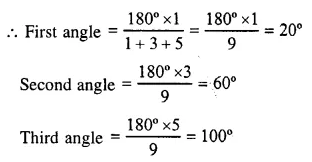## RS Aggarwal Class 6 Solutions Chapter 16 Triangles Ex 16A

These Solutions are part of RS Aggarwal Solutions Class 6. Here we have given RS Aggarwal Solutions Class 6 Chapter 16 Triangles Ex 16A

Other Exercises

Question 1.
Solution:
A, B and C are three non-collinear points in a plane. AB, BC and CA are joined.(i) The side opposite ∠C is AB
(ii) The angle opposite to the side BC is ∠A
(iii) The vertex opposite to the side CA is B
(iv) The side opposite to the vertex B is CA

Question 2.
Solution:
The measures of two angles of a triangle are 72° and 58°
But measure of three angles of a triangle is 180°
Third angle will be = 180 – (72° + 58°)
= 180° – 130°
= 50°

Question 3.
Solution:
Sum of three angles of a triangle = 180°
Ratio of three angles = 1 : 3 : 5Hence, three angles are 20°, 60° and 100°
Ans.

Question 4.
Solution:
Sum of three angles of a right triangle = 180°
Sum of two acute angles = 180°- 90°
= 90°
Measure of one angle = 50°
Second acute angle = 90° – 50° = 40°
Ans.

Question 5.
Solution:
Let the measure of each of the equal angles be x°. Then,
x° + x°+ 110°= 180°
(Angle sum property of a triangle)
=> 2x°+110°= 180°
=> 2x° = 180° – 110° = 70°
=> $${ x }^{ O }={ \left( \frac { 70 }{ 2 } \right) }^{ O }={ 35 }^{ O }$$
The measure of each of the equal angles is 35°.

Question 6.
Solution:
Let the three angles of a triangle be ∠A, ∠B, ∠C. Then, ∠A = ∠B + ∠C
Adding ∠A to both sides, we get ∠A + ∠A = ∠A + ∠B + ∠C
=> 2 ∠A = 180°
(Angle sum property of a triangle)
=> ∠A = $${ \left( \frac { 180 }{ 2 } \right) }^{ O }={ 90 }^{ O }$$
One of the angles of the triangle is a right angle.
Hence, the triangle is a right triangle

Question 7.
Solution:
In a ∆ABC,
3∠A = 4∠B = 6∠C = 1 (say)Question 8.
Solution:
(i) It is obtuse triangle.
(ii) It is acute triangle.
(iii) It is right triangle.
(iv) It is obtuse triangle.

Question 9.
Solution:
(i) It is an isosceles triangle as it has two equal sides.
(ii) It is an isosceles triangle as it has two equal sides.
(iii) It is a scalene triangle as its sides are different in length.
(iv) It is an equilateral triangle as its all sides are equal.
(v) It is an equilateral triangles as its angles are equal, so its sides will also be equal.
(vi) It is an isosceles triangle as its two base angles are equal, so its two sides are equal.
(vii) It is a scalene triangle as its angles are different, so its sides will also be different or unequal.

Question 10.
Solution:
In ∆ABC, D is a point on BC and AD is joined
Now we get triangles ∆ABC, ∆ABD and ∆ADC

Question 11.
Solution:
(i) No
(ii) No
(iii) Yes
(iv) No
(v) No
(vi) Yes.

Question 12.
Solution:
(i) three, three, three.
(ii) 180°
(iii) different
(iv) 60°
(v) equal
(vi) perimeter.

Hope given RS Aggarwal Solutions Class 6 Chapter 16 Triangles Ex 16A are helpful to complete your math homework.

If you have any doubts, please comment below. Learn Insta try to provide online math tutoring for you.